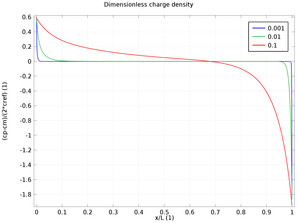# Galleria delle Applicazioni

## Diffuse Double Layer with Charge Transfer

Application ID: 13525

In the diffuse double layer and within the first few nanometers of an electrode surface, the assumption of electroneutrality is not valid due to charge separation. Typically, the diffuse double layer may be of interest when modeling very thin layers of electrolyte including those in electrochemical capacitors and microelectrodes. This example shows how to couple the Nernst-Planck equations to Poisson’s equation, in order to consider this deviation from electroneutrality. A Stern layer with constant capacity is used to derive surface charge boundary conditions for Poisson’s equation. This 1D model reproduces the results published in literature.This model example illustrates applications of this type that would nominally be built using the following products: